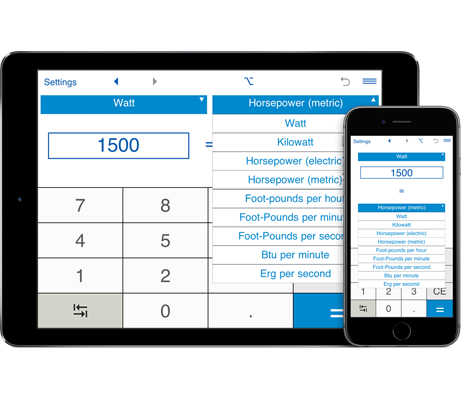# Power Units Converter

Power Converter is an application that lets you convert between US, imperial and metric power units: watts (w), kilowatts (kw), electric horsepower hp(E), metric horsepower hp(M), foot-pounds per hour (ft-lbf/h), foot-pounds per minute (ft-lbf/min), foot-pounds per second (ft-lbf/s), btu per minute (btu/min), and erg per second (erg/s).

## Power converter

You can use the power conversion calculator to convert between US, imperial and metric power units. For example, you could convert from kilowatts to horsepower, from electric horsepower to watts, etc.

"Flip" button lets you easily switch between the "from" and "to" units.

Power converter supports fractional values.

Results are shown in the decimal and fractional forms.

Power unit converter settings:
- Rounding to the specified number of decimal places: for decimal numbers, you can select the number of decimal places you want to display. By default, the app displays 2 decimal places.
To round results to the nearest whole number, select 0 decimal places.

- For fractions, you can select rounding to the nearest 1/2, 1/4, 1/8, 1/16, 1/32, 1/64, 1/128, 1/256. By default, the app rounds fractions to the nearest 1/32.
If you do not need to round fractions, just select "-".